# Practice Questions with Solutions: Fractions Notes | Study Mathematics for Class 4 - Class 4

## Class 4: Practice Questions with Solutions: Fractions Notes | Study Mathematics for Class 4 - Class 4

The document Practice Questions with Solutions: Fractions Notes | Study Mathematics for Class 4 - Class 4 is a part of the Class 4 Course Mathematics for Class 4.
All you need of Class 4 at this link: Class 4

Question 1: Write next three equivalent fractions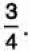We have: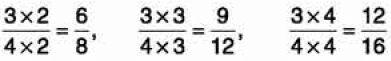So,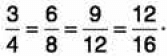Hence, the equivalent fractions to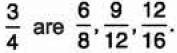Question 2: Find the sum of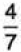and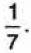We have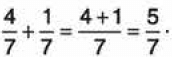Question 3: Subtract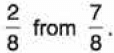We have :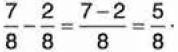Question 4: Express each of the following fractions to its lowest terms :
(a)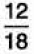(b)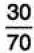(a) We have,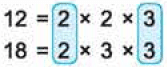So, we can divide the numerator and the denominator by 2 x 3 = 6.
So,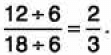Thus, the simplest form of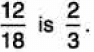(b) We have,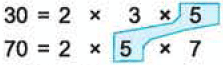So, we can divide the numerator and the denominator by 2 x 5 = 10 .
So,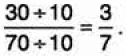Thus, the Simplest form of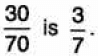Question 5: Subtract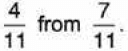We have: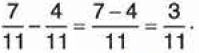Question 6: Find the sum of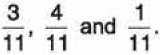We have :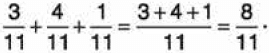Question 7: Compare :
(a)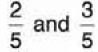(b)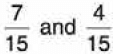We have:
(a) Consider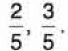Since, 3 > 2, hence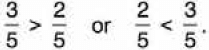(b) Consider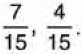Since, 7 > 4, hence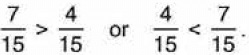Question 8: Find an equivalent fraction of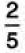with numerator 8.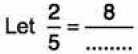To get 8 in the numerator, we multiply 2 by 4, and also 5 by 4.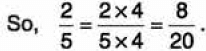Hence,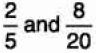are equivalent fractions.

Question 9: Reduce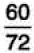to its lowest terms.

We can divide the numerator and the denominator step by step, by their common factors.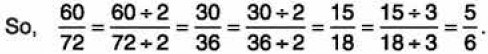Thus,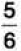is in its lowest terms.

Question 10: Find an equivalent fraction of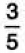with denominator 15.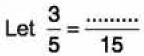To get 15 in the denominator, we multiply 5 by 3 and also 3 by 3.
So,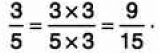Hence,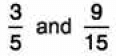are equivalent fractions.

Question 11Are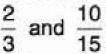equivalent fractions?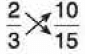2 X 15 = 30, 3 X 10 = 30
Both the products are equal.
Hence,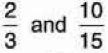are equivalent fractions.

Question 12: Are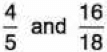equivalent fractions?
Solution: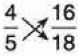Here, 4 x 18 = 72, 5 x 16 = 80
Products are not the same.
Hence,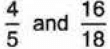are not equivalent fractions.

Question 13: Compare
(a)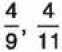(b)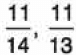(a)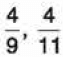Since 9 < 11.
Hence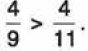(b)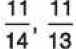Since 14 > 13
Hence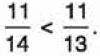Question 14: Compare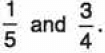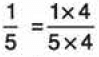[Multiplying the numerator and the denominator by 4]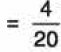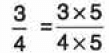[Multiplying the numerator and the denominator by 5]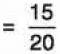Clearly,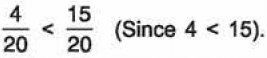Hence,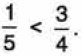Another method: Two fractions can be compared by using the method of cross multiplication.

Question 15: Compare
(a)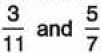(b)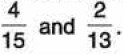(a) Given fractions are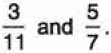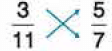(Cross multiply)
Now 3 x 7 = 21 and 11 x 5 = 55.
Since 21 < 55, hence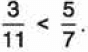(b) Given fractions are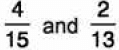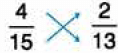(Cross multiply)
Now 4 x 13 = 52 and 15 x 2 = 30.
Since, 52 > 30, hence,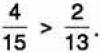The document Practice Questions with Solutions: Fractions Notes | Study Mathematics for Class 4 - Class 4 is a part of the Class 4 Course Mathematics for Class 4.
All you need of Class 4 at this link: Class 4Use Code STAYHOME200 and get INR 200 additional OFF

## Mathematics for Class 4

15 videos|68 docs|32 tests

Track your progress, build streaks, highlight & save important lessons and more!

,

,

,

,

,

,

,

,

,

,

,

,

,

,

,

,

,

,

,

,

,

;